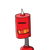# calculate the amount and compound interest on rs.10800 for 3 year’s at 12.5% per annum compound anually​

calculate the amount and compound interest on rs.10800 for 3 year’s at 12.5% per annum compound anually​

### 2 thoughts on “calculate the amount and compound interest on rs.10800 for 3 year’s at 12.5% per annum compound anually​”

1.## Question:-

• Calculate the amount and compound interest on rs.10800 for 3 year’s at 12.5% per annum compound anually.

## To Find:-

• Find the amount and the compound interest.

## Solution:-

$$\tt\implies \: A = 10800 \times { ( \dfrac { 1 + 12.5 } { 100 } ) }^{ 3 }$$

$$\tt\implies \: A = 10800 \times {1.125 }^{ 3 }$$

$$\tt\implies \: A = 15377$$

Now ,

We have to find the compound Interest:-

$$\tt\implies \: Interest = 15377 – 10800$$

$$\tt\implies \: Interest = 4577$$

Hence ,

• Compound interest is 4577
2.1. Amount = Rs 15377
2. Compound interest = Rs 4577

Step-by-step explanation:

Given that:

• Time = 3 years
• Principal = Rs 10800
• Rate of interest = 12.5% per annum

To Find:

1. Amount
2. Compound interest

Formula used:

1. A = P(1 + R/100)ᵀ
2. CI = A – P

Where,

• P = Principal
• R = Rate of interest
• T = Time
• A = amount
• CI = Compound interest

Finding the amount:

⟶ A = 10800(1 + 12.5/100)³

⟶ A = 10800(1 + 0.125)³

⟶ A = 10800(1.125)³

⟶ A = 10800 × 1.125 × 1.125 × 1.125

⟶ A ≈ 15377

∴ Amount in compound interest = Rs 15377

Finding the compound interest:

⟶ CI = 15377 – 10800

⟶ CI = 4577

∴ Compound interest = Rs 4577